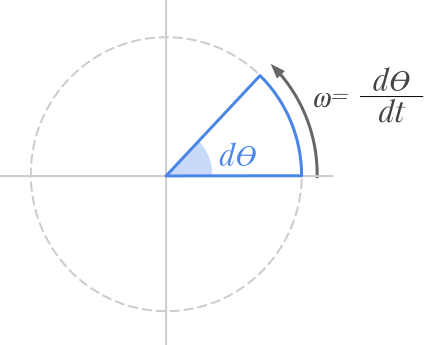Question #570

# What is the formula of angular velocity?

Merged questions
Answer

Angular velocity refers to how fast an object rotates with respect to time, i.e. an object’s angular displacement with respect to time.The general formula of the angular velocity is defined by the derivative of the angular position with respect to time:

﻿﻿

Where :

• ﻿﻿ is the angular velocity expressed in radians per second ( ﻿﻿ )
• ﻿﻿ refers to the angular position expressed in radians ( ﻿﻿ )
• ﻿﻿ refers to the time expressed in seconds ( ﻿﻿ )

﻿﻿ can viewed as the elementary angular displacement with respect to its duration (﻿﻿).

6 events in history
Answer by Alphonsio 01/01/2021 at 05:37:21 PM

Angular velocity refers to how fast an object rotates with respect to time, i.e. an object’s angular displacement with respect to time.The general formula of the angular velocity is defined by the derivative of the angular position with respect to time:

﻿﻿

Where :

• ﻿﻿ is the angular velocity expressed in radians per second ( ﻿﻿ )
• ﻿﻿ refers to the angular position expressed in radians ( ﻿﻿ )
• ﻿﻿ refers to the time expressed in seconds ( ﻿﻿ )

﻿﻿ can viewed as the elementary angular displacement with respect to its duration (﻿﻿).

Answer by Alphonsio 01/01/2021 at 05:23:04 PM

Angular velocity refers to how fast an object rotates with respect to time, i.e. an object’s angular displacement with respect to time.The general formula of the angular velocity is defined by the derivative of the angular position with respect to time:

﻿﻿

Where :

• ﻿﻿ is the angular velocity expressed in radians per second ( ﻿﻿ )
• ﻿﻿ refers to the angular position expressed in radians ( ﻿﻿ )
• ﻿﻿ refers to the time expressed in seconds ( ﻿﻿ )

﻿﻿ can viewed as the elementary angular displacement with respect to its duration (﻿﻿).

Answer by Alphonsio 01/01/2021 at 05:22:55 PM

Angular velocity refers to how fast an object rotates with respect to time, i.e. an object’s angular displacement with respect to time.The general formula of the angular velocity is defined by the derivative of the angular position with respect to time:

﻿﻿

Where :

• ﻿﻿ is the angular velocity expressed in radians per second ( ﻿﻿ )
• ﻿﻿ refers to the angular position expressed in radians ( ﻿﻿ )
• ﻿﻿ refers to the time expressed in seconds ( ﻿﻿ )

﻿﻿ can viewed as the elementary angular displacement with respect to its duration (﻿﻿).

Answer by Alphonsio 01/01/2021 at 05:22:13 PM

Angular velocity refers to how fast an object rotates with time, i.e. an object’s angular displacement with respect to time.The general formula of the angular velocity is defined by the derivative of the angular position with respect to time:

﻿﻿

Where :

• ﻿﻿ is the angular velocity expressed in radians per second ( ﻿﻿ )
• ﻿﻿ refers to the angular position expressed in radians ( ﻿﻿ )
• ﻿﻿ refers to the time expressed in seconds ( ﻿﻿ )

﻿﻿ can viewed as the elementary angular displacement with respect to its duration (﻿﻿).

Answer by Alphonsio 01/01/2021 at 05:18:57 PM

Angular velocity refers to how fast an object rotates with time, i.e. an object’s angular displacement with respect to time.The general formula of the angular velocity is defined by the derivative of the angular position with respect to time:

﻿﻿

Where :

• ﻿﻿ is the angular velocity expressed in radians per second ( ﻿﻿ )
• ﻿﻿ refers to the angular position expressed in radians ( ﻿﻿ )
• ﻿﻿ refers to the time expressed in seconds ( ﻿﻿ )

Note that ﻿﻿ can be viewed as the elementary angular displacement with respect to its duration (﻿﻿).

Question by Alphonsio 01/01/2021 at 05:06:07 PM
What is the formula of angular velocity?

Icons proudly provided by Friconix.